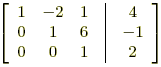index: click on a letter A B C D E F G H I J K L M N O P Q R S T U V W X Y Z A to Z index index: subject areas numbers & symbols sets, logic, proofs geometry algebra trigonometry advanced algebra & pre-calculus calculus advanced topics probability & statistics real world applications multimedia entrieswww.mathwords.com about mathwords website feedback

Back-Substitution

The process of solving a linear system of equations that has been transformed into row-echelon form or reduced row-echelon form. The last equation is solved first, then the next-to-last, etc.

 Example: Consider a system with the given row-echelon form for its augmented matrix.The equations for this system are \eqalign{x - 2y + z &= 4\\y + 6z &= - 1\\z &= 2} The last equation says z = 2. Substitute this into the second equation to get \eqalign{y + 6\left( 2 \right) &= - 1\\y &= - 13} Now substitute z = 2 and y = –13 into the first equation to get \eqalign{x - 2\left( { - 13} \right) + \left( 2 \right) &= 4\\x &= - 24} Thus the solution is x = –24, y = –13, and z = 2.